## Classical Scalar Field in Four Dimensions

Assume we have a field defined everywhere in space and time. For simplicity we will start with a scalar field(instead of the vector... fields of E&M).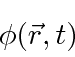The property that makes this a true scalar field is that it is invariant under rotations and Lorentz boosts.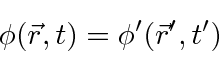The Euler-Lagrange equation derived from the principle of least action isNote that since there is only one field, there is only one equation.

Since we are aiming for a description of relativistic quantum mechanics, it will benefit us to write our equations in a covariant way. I think this also simplifies the equations. We will follow the notation of Sakurai. (The convention does not really matter and one should not get hung up on it.) As usual the Latin indices likewill run from 1 to 3 and represent the space coordinates. The Greek indices likewill run from 1 to 4. Sakurai would give the spacetime coordinate vector either asor asand use the former to do real computations.

We will not use the so called covariant and contravariant indices. Instead we will put anon the fourth component of a vector which give that component asign in a dot product.Note we can have all lower indices. As Sakurai points out, there is no need for the complication of a metric tensor to raise and lower indices unless general relativity comes into play and the geometry of space-time is not flat. We can assume thein the fourth component is a calculational convenience, not an indication of the need for complex numbers in our coordinate systems. So while we may have said farewell to ict'' some time in the past, we will use it here because the notation is less complicated. Thehere should never really be used to multiply anin the complex wave function, but, everything will work out so that doesn't happen unless we make an algebra mistake.

The spacetime coordinate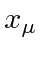is a Lorentz vector transforming under rotations and boosts as follows.(Note that we will always sum over repeated indices, Latin or Greek.) The Lorentz transformation is done with a 4 by 4 matrix with the property that the inverse is the transpose of the matrix.Theand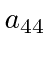are real while the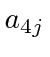andare imaginary in our convention. Thus we may compute the coordinate using the inverse transformation.Vectors transform as we change our reference system by rotating or boosting. Higher rank tensors also transform with one Lorentz transformation matrix per index on the tensor.

The Lorentz transformation matrix to a coordinate system boosted along the x direction is.Theshows up on space-time elements to deal with thewe have put on the time components of 4-vectors. It is interesting to note the similarity between Lorentz boosts and rotations. A rotation in the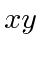plane through an angleis implemented with the transformationA boost along thedirection is like a rotation in thethrough an angle ofwhere. Since we are in Minkowski space where we need a minus sign on the time component of dot products, we need to add anin this rotation too.Effectively, a Lorentz boost is a rotation in which. We will make essentially no use of Lorentz transformations because we will write our theories in terms of Lorentz scalarswhenever possible. For example, our Lagrangian density should be invariant.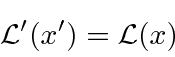The Lagrangians we have seen so far have derivatives with respect to the coordinates. The 4-vector way of writing this will be. We need to know what the transformation properties of this are. We can compute this from the transformations and the chain rule.This means that it transforms like a vector. Compare it to our original transformation formula for.We may safely assume that all our derivatives with one index transform as a vector.

With this, lets work on the Euler-Lagrange equation to get it into covariant shape. Remember that the fieldis a Lorentz scalar.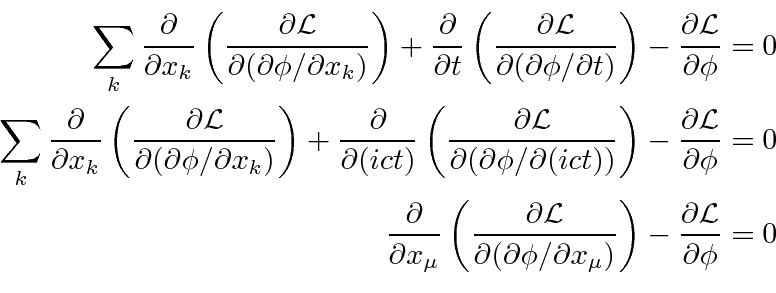This is the Euler-Lagrange equation for a single scalar field. Each term in this equation is a Lorentz scalar, ifis a scalar.Now we want to find a reasonable Lagrangian for a scalar field. The Lagrangian depends on theand its derivatives. It should not depend explicitly on the coordinates, since that would violate translation and/or rotation invariance. We also want to come out with a linear wave equation so that high powers of the field should not appear. The only Lagrangian we can choose (up to unimportant constants) is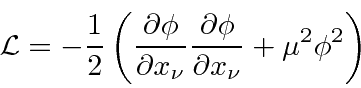The one constant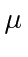sets the ratio of the two terms. The overall constant is not important except to match thedefinition. Remember thatis a function of the coordinates.

With this Lagrangian, the Euler-Lagrange equation is.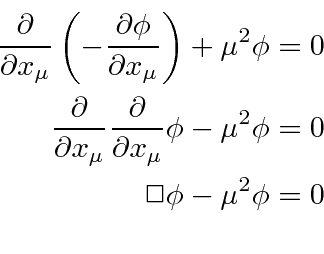This is the known as the Klein-Gordon equation. It is a good relativistic equation for a massive scalar field. It was also an early candidate for the relativistic equivalent of the Schrödinger equation for electrons because it basically has the relativistic analog of the energy relation inherent in the Schrödinger equation. Writing that relation in the order terms appear in the Klein-Gordon equation above we get (lettingbriefly).Its worth noting that this equation, unlike the non-relativistic Schrödinger equation, relates the second spatial derivative of the field to the second time derivative. (Remember the Schrödinger equation hastimes the first time derivative as the energy operator.)

So far we have the Lagrangian and wave equation for a free'' scalar field. There are no sources of the field (the equivalent of charges and currents in electromagnetism.) Lets assume the source density is. The source term must be a scalar function so, we add the termto the Lagrangian.This adds a term to the wave equation.Any source density can be built up from point sources so it is useful to understand the field generated by a point source as we do for electromagnetism.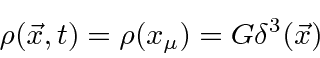This is a source of strengthat the origin. It does not change with time so we expect a static field.The Euler-Lagrange equation becomes.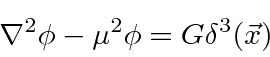We will solve this for the field from a point source below and get the resultThis solution should be familiar to us from the scalar potential for an electric point charge which satisfies the same equation with,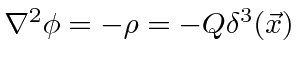. This is a field that falls off much faster than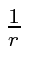. A massive scalar field falls off exponentially and the larger the mass, the faster the fall off. (We also get a mathematical result which is useful in several applications. This is worked out another way in the section on hyperfine splitting)Now we solve for the scalar field from a point source by Fourier transforming the wave equation. Define the Fourier transforms to be.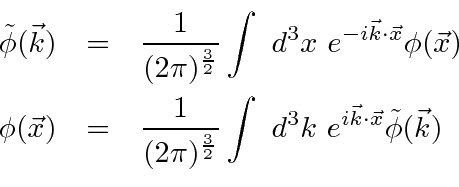We now take the transform of both sides of the equation.To deal with the, we have integrated by parts twice assuming that the field falls off fast enough at infinity.

We now have the Fourier transform of the field of a point source. If we can transform back to position space, we will have the field. This is a fairly standard type of problem in quantum mechanics.This is now of a form for which we can use Cauchy's theorem for contour integrals. The theorem says that an integral around a closed contour in the complex plane is equal totimes the sum of the residues at the poles enclosed in the contour. Contour Integration is a powerful technique often used in quantum mechanics.

If the integrand is a function of the complex variable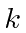, a pole is of the form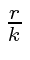whereis called the residue at the pole. The integrand above has two poles, one atand the other at. The integral we are interested in is just along the real axis so we want the integral along the rest of the contour to give zero. That's easy to do since the integrand goes to zero at infinity on the real axis and the exponentials go to zero either at positive or negative infinity for the imaginary part of. Examine the integral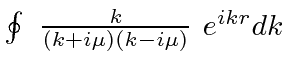around a contour in the upper half plane as shown below.The pole inside the contour is at. The residue at the pole isThe integrand goes to zero exponentially on the semicircle at infinity so only the real axis contributes to the integral along the contour. The integral along the real axis can be manipulated to do the whole problem for us.This is exactly the integral we wanted to do.

Plug the integral into the Fourier transform we were computing.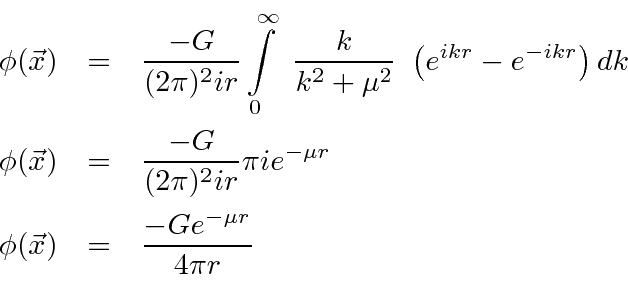In this case, it is simple to compute the interaction Hamiltonian from the interaction Lagrangian and the potential between two particles. Lets assume we have two particles, each with the same interaction with the field.Now compute the Hamiltonian.We see that this is a short-range, attractive potential.

This was proposed by Yukawa as the nuclear force. He predicted a scalar particle with a mass close to that of the pion before the pion was discovered. His prediction of the mass was based on the range of the nuclear force, on the order of one Fermi. In some sense, his prediction is approximately correct. Pion exchange can explain much of the nuclear force but does not explain all the details. Pions and nucleons have since been show to be composite particles with internal structure. Other composites with masses larger than the pion also play a role in the force between nucleons.

Pions were also found to come in three charges:,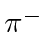, and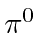. This would lead us to develop a complex scalar field as done in the text. Its not our goal right now so we will skip this. Its interesting to note that the Higgs Boson is also represented by a complex scalar field.

We have developed a covariant classical theory for a scalar field. The Lagrangian density is a Lorentz scalar function. We have included an interaction term to provide a source for the field. Now we will attempt to do the same for classical electromagnetism.

Jim Branson 2013-04-22